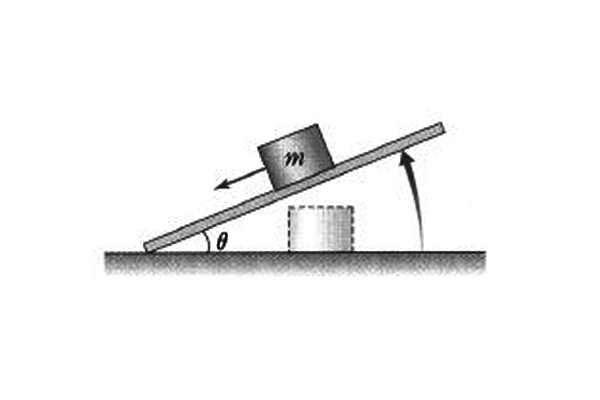# Inclined planeIn the above figure, the angle $\theta$ between the inclined plane and the floor is being increased gradually, until the object with mass m starts to slide down the slope at $\theta = 45°.$ Find the coefficient of static friction between the plane and the object.

Gravitational acceleration is $g= 10$ m/s$^{2}.$

×